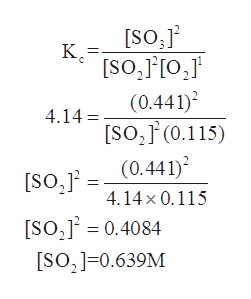# An equilibrium mixture of the following reaction was found to have [SO3]= 0.441 M and [O2]= 0.115 M at 600 ∘C.What is the concentration of SO2?2SO2(g)+O2(g)⇌2SO3(g)Keq= 4.14 at 600 ∘C

Question
9 views

An equilibrium mixture of the following reaction was found to have [SO3]= 0.441 M and [O2]= 0.115 M at 600 ∘C.

What is the concentration of SO2?
2SO2(g)+O2(g)⇌2SO3(g)
Keq= 4.14 at 600 ∘C

check_circle

Step 1

The Equilibrium constant for a given reaction can be defined as the relation between the reactants and the products that have reached equilibrium to some specific unit. For a hypothetical reaction, say,

Step 2

The given reaction is as follows:

Step 3

The concentration of SO3 gas is given to be 0.441 M.

The concentration of O2 gas is given to be 0.115 M.

The equilibrium constant at a give...help_outlineImage Transcriptionclose[SO,j2 К [SO, [O, (0.441) 4.14 [SO,F (0.115) (0.441) [SO,j2 4.14x0.115 [So, 0.4084 SO,0.639M fullscreen

### Want to see the full answer?

See Solution

#### Want to see this answer and more?

Solutions are written by subject experts who are available 24/7. Questions are typically answered within 1 hour.*

See Solution
*Response times may vary by subject and question.
Tagged in

### Physical Chemistry